hist

Histogram plot (not recommended; use histogram)

hist is not recommended. Use histogram instead.

For more information, including suggestions on updating code, see Replace Discouraged Instances of hist and histc.

Description

example

hist(x) creates a histogram bar chart of the elements in vector x. The elements in x are sorted into 10 equally spaced bins along the x-axis between the minimum and maximum values of x. hist displays bins as rectangles, such that the height of each rectangle indicates the number of elements in the bin.

If the input is a multi-column array, hist creates histograms for each column of x and overlays them onto a single plot.

If the input is of data type categorical, each bin is a category of x.

example

hist(x,nbins) sorts x into the number of bins specified by the scalar nbins.

hist(x,xbins) sorts x into bins with intervals or categories determined by the vector xbins.

• If xbins is a vector of evenly spaced values, then hist uses the values as the bin centers.

• If xbins is a vector of unevenly spaced values, then hist uses the midpoints between consecutive values as the bin edges.

• If x is of data type categorical, then xbins must be a categorical vector or cell array of character vectors that specifies categories. hist plots bars only for those categories.

The length of the vector xbins is equal to the number of bins.

hist(ax,___) plots into the axes specified by ax instead of into the current axes (gca). The option ax can precede any of the input argument combinations in the previous syntaxes.

counts = hist(___) returns a row vector, counts, containing the number of elements in each bin.

example

[counts,centers] = hist(___) returns an additional row vector, centers, indicating the location of each bin center on the x-axis.

Examples

collapse all

x = [0 2 9 2 5 8 7 3 1 9 4 3 5 8 10 0 1 2 9 5 10];
hist(x)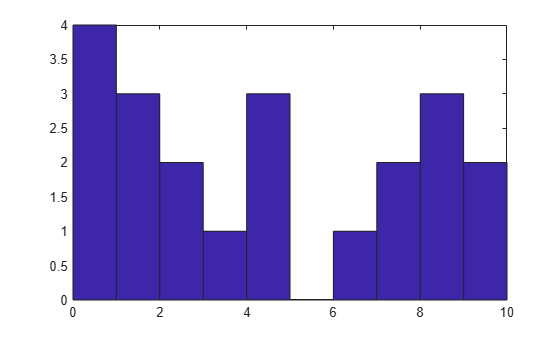hist sorts the values in x among 10 equally spaced bins between the minimum and maximum values in the vector, which are 0 and 10 in this example.

Generate three columns of 1,000 random numbers and plot the three column overlaid histogram.

x = randn(1000,3);
hist(x)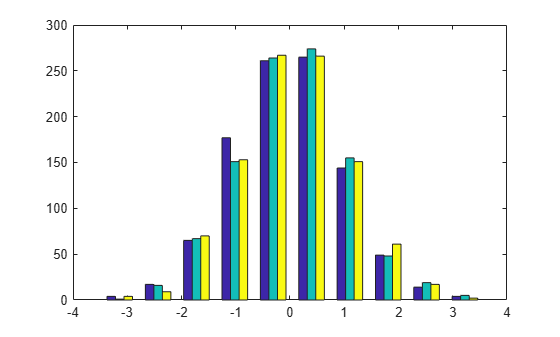The values in x are sorted among 10 equally spaced bins between the minimum and maximum values. hist sorts and bins the columns of x separately and plots each column with a different color.

Plot a histogram of 1,000 random numbers sorted into 50 equally spaced bins.

x = randn(1000,1);
nbins = 50;
hist(x,nbins)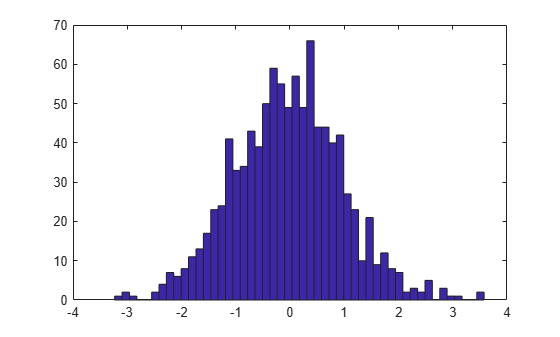Generate 1,000 random numbers. Count how many numbers are in each of 10 equally spaced bins. Return the bin counts and bin centers.

x = randn(1000,1);
[counts,centers] = hist(x)
counts = 1×10

4    27    88   190   270   243   123    38    13     4

centers = 1×10

-2.8915   -2.2105   -1.5294   -0.8484   -0.1673    0.5137    1.1947    1.8758    2.5568    3.2379

Use bar to plot the histogram.

bar(centers,counts)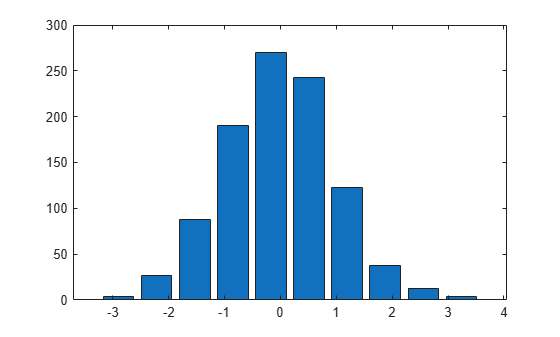Generate 1,000 random numbers and create a histogram.

data = randn(1000,1);
hist(data)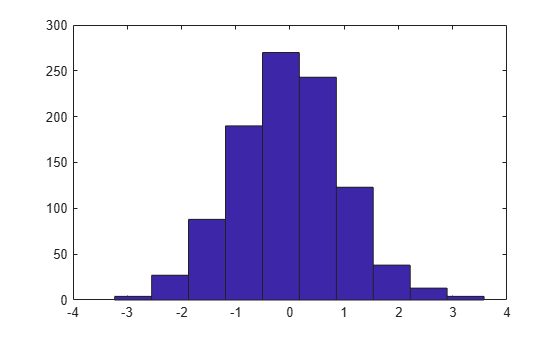Get the handle to the patch object that creates the histogram plot.

h = findobj(gca,'Type','patch');

Set the face color of the bars plotted to an RGB triplet value of [0 0.5 0.5]. Set the edge color to white.

h.FaceColor = [0 0.5 0.5];
h.EdgeColor = 'w';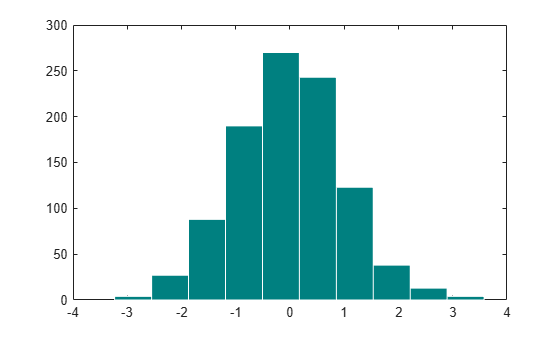Input Arguments

collapse all

Input vector or matrix.

• If x is a vector, then hist creates one histogram.

• If x is a matrix, then hist creates a separate histogram for each column and plots the histograms using different colors.

If the input array contains NaNs or undefined categorical values, hist does not include these values in the bin counts.

If the input array contains the infinite values -Inf or Inf, then hist sorts -Inf into the first bin and Inf into the last bin. If you do not specify the bin intervals, then hist calculates the bin intervals using only the finite values in the input array.

Data Types: single|double|logical|categorical

Number of bins. Input x must be numeric, not categorical.

Data Types: single|double|int8|int16|int32|int64|uint8|uint16|uint32|uint64

Bin locations or categories, specified as a vector.

If x is numeric or logical, then xbins must be of type single or double.

• If the elements in xbins are equally spaced, then these elements are the bin centers.

• If the elements in xbins are not equally spaced, then these elements are indicated by markers along the x-axis, but are not the actual bin centers. Instead, hist calculates the bin edges as the midpoints between consecutive elements in vector xbins. To specify the bin edges directly, use histc.

• xbins must contain only finite values. The first and last bins extend to cover the minimum and maximum values in x.

If x is categorical, then xbins must be a categorical vector or cell array of character vectors that specifies categories. hist plots bars only for those categories specified by xbins.

Axes object. Use ax to plot the histogram in a specific axes instead of the current axes (gca).

Output Arguments

collapse all

Counts of the number of elements in each bin, returned as a row vector.

Bin centers or categories, returned as a vector. If used with the syntax [counts,centers] = hist(x,xbins), then the centers output has the same elements as the xbins input.

• If x is numeric or logical, then centers is a numeric row vector.

• If x is categorical, then centers is a cell array of character vectors.